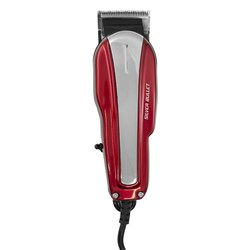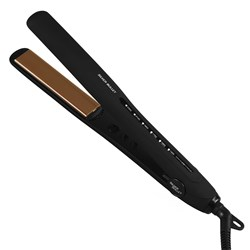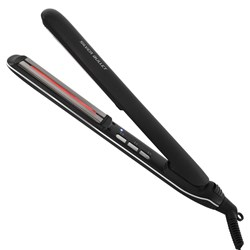# Search Results

There were no products that contained all of the words in your query. These products contain some of the words:
Grid List
Increase value Decrease value
Increase value Decrease value
Silver Bullet Super Pod Hot Roller Heater \$199.95 Now \$99.98 Save 50%
Increase value Decrease value
Increase value Decrease valueIncrease value Decrease value
Increase value Decrease value
Increase value Decrease value
Increase value Decrease value
Increase value Decrease value
Increase value Decrease value
Increase value Decrease value
Increase value Decrease value
Increase value Decrease value
Increase value Decrease value
Increase value Decrease value
Increase value Decrease value
Increase value Decrease valueIncrease value Decrease value
Increase value Decrease value
Increase value Decrease value
Increase value Decrease value
Increase value Decrease value
Silver Bullet Thermal Hot Roller Set 12pc \$129.95 Now \$77.97 Save 40%
Increase value Decrease valueIncrease value Decrease value
Grid List